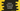# How to use Python numpy.all method with examples## How to use numpy.all method with examples:

In this post, we will learn how to use the all method of NumPy with an example. This method is used to test if the elements of an array returns True along an axis.

In this post, we will learn how to use numpy.all method and its definition with an example.

### Definition of numpy.all:

numpy.all method is defined as like below:

``numpy.all(a, axis=None, out=None, keepdims=<no value>, *, where=<no value>)``

Here,

• a is the input array of any other object which can be converted to an array.
• axis can be one int or tuple of ints or None. This is an optional value. It defines the axis or axes along which AND is performed. If we don’t provide any value to this parameter, the AND is performed over all the dimensions of the input array.
• out is an optional value. It can be a ndarray. It will hold the output if provided. It should have the same output as the result we are expecting.
• keepdims is an optional boolean value. If we pass True, the axes which are reduced are left in the result as dimensions with size one.
• where is another optional parameter. It is array_like of bool. These are the elements to include while checking for True values.

#### Return value:

It returns a boolean value or an array. If out is defined, it returns a reference to out.

## Example of numpy.all:

Let me show you how it works with different examples:

``````import numpy as np

print(f'all(0) => {np.all(0)}')
print(f'all(1) => {np.all(1)}')
print(f'all([0,1]) => {np.all([0,1])}')
print(f'all([[0,0], [1,1]], axis=0) => {np.all([[0,0], [1,1]], axis=0)}')
print(f'all([[0,0], [1,1]], axis=1) => {np.all([[0,0], [1,1]], axis=1)}')
print(f'all(nan) => {np.all(np.nan)}')

given_arr = np.array([1, 2, 3, 4, 5])
even_arr = np.array([2, 4, 6, 8, 10])

print(f'all(given_arr%2 == 0) => {np.all(given_arr%2 == 0)}')
print(f'all(even_arr%2 == 0) => {np.all(even_arr%2 == 0)}')``````

In this example, I am showing how to use numpy.all with different types of parameters. If you run this program, it will print the below output:

``````all(0) => False
all(1) => True
all([0,1]) => False
all([[0,0], [1,1]], axis=0) => [False False]
all([[0,0], [1,1]], axis=1) => [False  True]
all(nan) => True
all(given_arr%2 == 0) => False
all(even_arr%2 == 0) => True``````

official doc